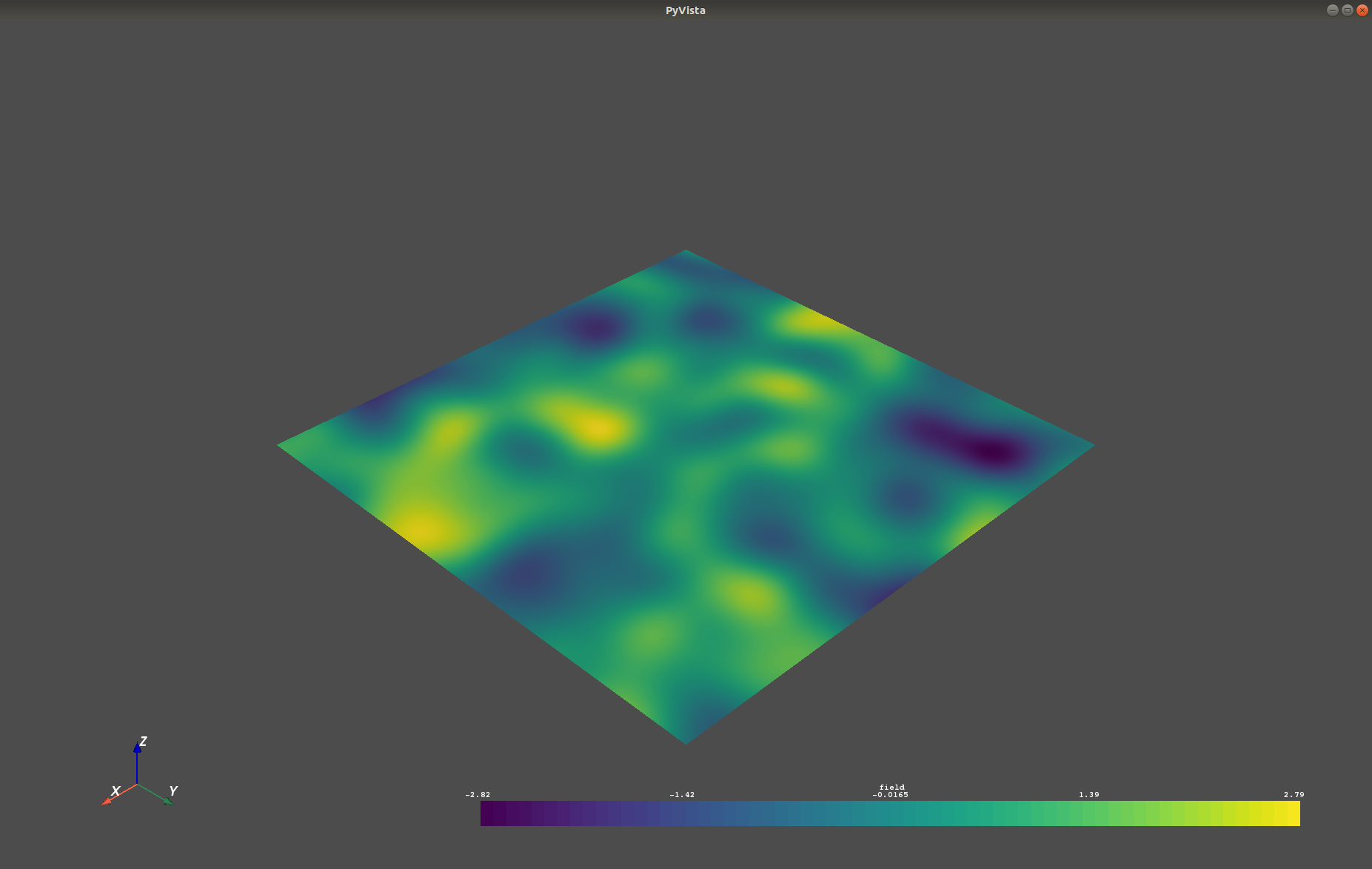# GSTools Quickstart¶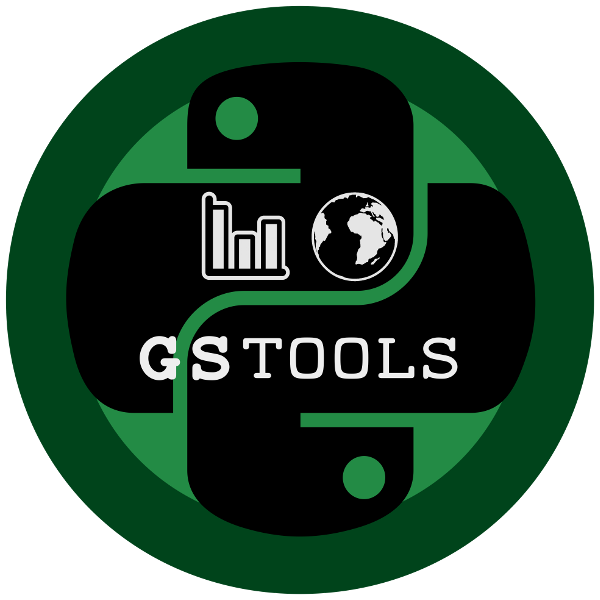Get in Touch!

## Purpose¶

GeoStatTools provides geostatistical tools for various purposes:

• random field generation

• simple, ordinary, universal and external drift kriging

• conditioned field generation

• incompressible random vector field generation

• (automated) variogram estimation and fitting

• directional variogram estimation and modelling

• data normalization and transformation

• many readily provided and even user-defined covariance models

• metric spatio-temporal modelling

• plotting and exporting routines

## Installation¶

### conda¶

GSTools can be installed via conda on Linux, Mac, and Windows. Install the package by typing the following command in a command terminal:

```conda install gstools
```

In case conda forge is not set up for your system yet, see the easy to follow instructions on conda forge. Using conda, the parallelized version of GSTools should be installed.

### pip¶

GSTools can be installed via pip on Linux, Mac, and Windows. On Windows you can install WinPython to get Python and pip running. Install the package by typing the following into command in a command terminal:

```pip install gstools
```

To get the latest development version you can install it directly from GitHub:

```pip install git+git://github.com/GeoStat-Framework/GSTools.git@develop
```

If something went wrong during installation, try the `-I` flag from pip.

To enable the OpenMP support, you have to provide a C compiler and OpenMP. Parallel support is controlled by an environment variable `GSTOOLS_BUILD_PARALLEL`, that can be `0` or `1` (interpreted as `0` if not present). GSTools then needs to be installed from source:

```export GSTOOLS_BUILD_PARALLEL=1
pip install --no-binary=gstools gstools
```

Note, that the `--no-binary=gstools` option forces pip to not use a wheel for GSTools.

For the development version, you can do almost the same:

```export GSTOOLS_BUILD_PARALLEL=1
pip install git+git://github.com/GeoStat-Framework/GSTools.git@develop
```

## Citation¶

At the moment you can cite the Zenodo code publication of GSTools:

Sebastian Müller & Lennart Schüler. GeoStat-Framework/GSTools. Zenodo. https://doi.org/10.5281/zenodo.1313628

If you want to cite a specific version, have a look at the Zenodo site.

A publication for the GeoStat-Framework is in preperation.

## Tutorials and Examples¶

The documentation also includes some tutorials, showing the most important use cases of GSTools, which are

## Spatial Random Field Generation¶

The core of this library is the generation of spatial random fields. These fields are generated using the randomisation method, described by Heße et al. 2014.

### Examples¶

#### Gaussian Covariance Model¶

This is an example of how to generate a 2 dimensional spatial random field (`SRF`) with a `Gaussian` covariance model.

```import gstools as gs
# structured field with a size 100x100 and a grid-size of 1x1
x = y = range(100)
model = gs.Gaussian(dim=2, var=1, len_scale=10)
srf = gs.SRF(model)
srf((x, y), mesh_type='structured')
srf.plot()
```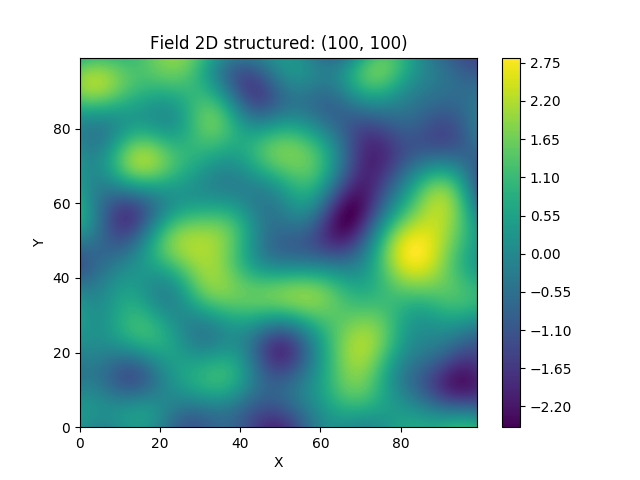GSTools also provides support for geographic coordinates. This works perfectly well with cartopy.

```import matplotlib.pyplot as plt
import cartopy.crs as ccrs
import gstools as gs
# define a structured field by latitude and longitude
lat = lon = range(-80, 81)
srf = gs.SRF(model, seed=12345)
field = srf.structured((lat, lon))
# Orthographic plotting with cartopy
ax = plt.subplot(projection=ccrs.Orthographic(-45, 45))
cont = ax.contourf(lon, lat, field, transform=ccrs.PlateCarree())
ax.coastlines()
ax.set_global()
plt.colorbar(cont)
```A similar example but for a three dimensional field is exported to a VTK file, which can be visualized with ParaView or PyVista in Python:

```import gstools as gs
# structured field with a size 100x100x100 and a grid-size of 1x1x1
x = y = z = range(100)
model = gs.Gaussian(dim=3, len_scale=[16, 8, 4], angles=(0.8, 0.4, 0.2))
srf = gs.SRF(model)
srf((x, y, z), mesh_type='structured')
srf.vtk_export('3d_field') # Save to a VTK file for ParaView

mesh = srf.to_pyvista() # Create a PyVista mesh for plotting in Python
mesh.contour(isosurfaces=8).plot()
```## Estimating and fitting variograms¶

The spatial structure of a field can be analyzed with the variogram, which contains the same information as the covariance function.

All covariance models can be used to fit given variogram data by a simple interface.

### Examples¶

This is an example of how to estimate the variogram of a 2 dimensional unstructured field and estimate the parameters of the covariance model again.

```import numpy as np
import gstools as gs
# generate a synthetic field with an exponential model
x = np.random.RandomState(19970221).rand(1000) * 100.
y = np.random.RandomState(20011012).rand(1000) * 100.
model = gs.Exponential(dim=2, var=2, len_scale=8)
srf = gs.SRF(model, mean=0, seed=19970221)
field = srf((x, y))
# estimate the variogram of the field
bin_center, gamma = gs.vario_estimate((x, y), field)
# fit the variogram with a stable model. (no nugget fitted)
fit_model = gs.Stable(dim=2)
fit_model.fit_variogram(bin_center, gamma, nugget=False)
# output
ax = fit_model.plot(x_max=max(bin_center))
ax.scatter(bin_center, gamma)
print(fit_model)
```

Which gives:

```Stable(dim=2, var=1.85, len_scale=7.42, nugget=0.0, anis=[1.0], angles=[0.0], alpha=1.09)
```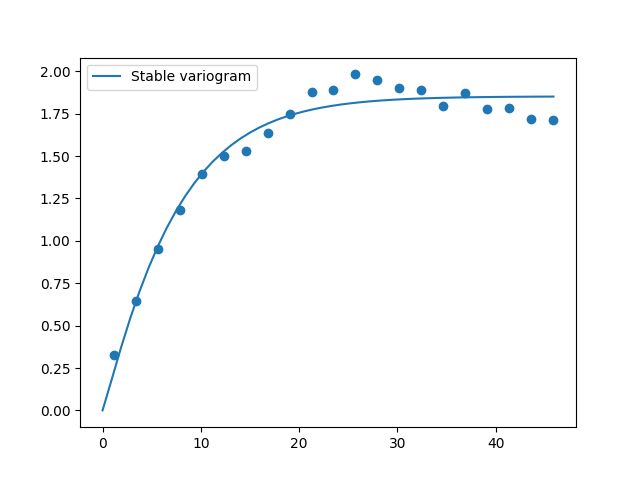## Kriging and Conditioned Random Fields¶

An important part of geostatistics is Kriging and conditioning spatial random fields to measurements. With conditioned random fields, an ensemble of field realizations with their variability depending on the proximity of the measurements can be generated.

### Example¶

For better visualization, we will condition a 1d field to a few “measurements”, generate 100 realizations and plot them:

```import numpy as np
import matplotlib.pyplot as plt
import gstools as gs

# conditions
cond_pos = [0.3, 1.9, 1.1, 3.3, 4.7]
cond_val = [0.47, 0.56, 0.74, 1.47, 1.74]

gridx = np.linspace(0.0, 15.0, 151)

# conditioned spatial random field class
model = gs.Gaussian(dim=1, var=0.5, len_scale=2)
krige = gs.krige.Ordinary(model, cond_pos, cond_val)
cond_srf = gs.CondSRF(krige)

# generate the ensemble of field realizations
fields = []
for i in range(100):
fields.append(cond_srf(gridx, seed=i))
plt.plot(gridx, fields[i], color="k", alpha=0.1)
plt.scatter(cond_pos, cond_val, color="k")
plt.show()
```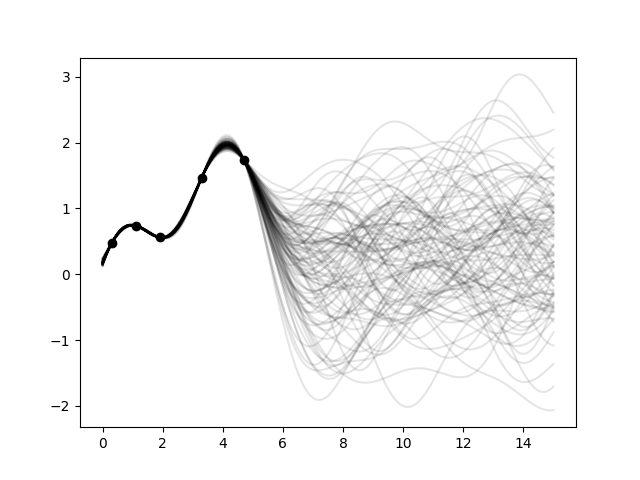## User defined covariance models¶

One of the core-features of GSTools is the powerful `CovModel` class, which allows to easy define covariance models by the user.

### Example¶

Here we re-implement the Gaussian covariance model by defining just the correlation function, which takes a non-dimensional distance `h = r/l`

```import numpy as np
import gstools as gs
# use CovModel as the base-class
class Gau(gs.CovModel):
def cor(self, h):
return np.exp(-h**2)
```

And that’s it! With `Gau` you now have a fully working covariance model, which you could use for field generation or variogram fitting as shown above.

## Incompressible Vector Field Generation¶

Using the original Kraichnan method, incompressible random spatial vector fields can be generated.

### Example¶

```import numpy as np
import gstools as gs
x = np.arange(100)
y = np.arange(100)
model = gs.Gaussian(dim=2, var=1, len_scale=10)
srf = gs.SRF(model, generator='VectorField', seed=19841203)
srf((x, y), mesh_type='structured')
srf.plot()
```

yielding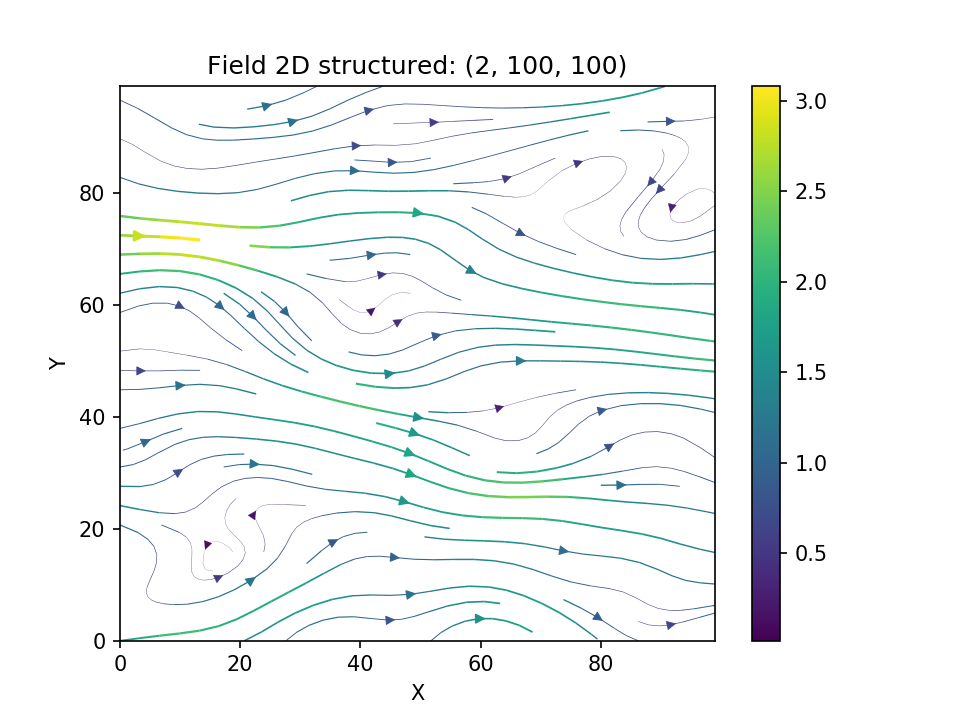## VTK/PyVista Export¶

After you have created a field, you may want to save it to file, so we provide a handy VTK export routine using the `vtk_export()` or you could create a VTK/PyVista dataset for use in Python with to `to_pyvista()` method:

```import gstools as gs
x = y = range(100)
model = gs.Gaussian(dim=2, var=1, len_scale=10)
srf = gs.SRF(model)
srf((x, y), mesh_type='structured')
srf.vtk_export("field") # Saves to a VTK file
mesh = srf.to_pyvista() # Create a VTK/PyVista dataset in memory
mesh.plot()
```

Which gives a RectilinearGrid VTK file `field.vtr` or creates a PyVista mesh in memory for immediate 3D plotting in Python.